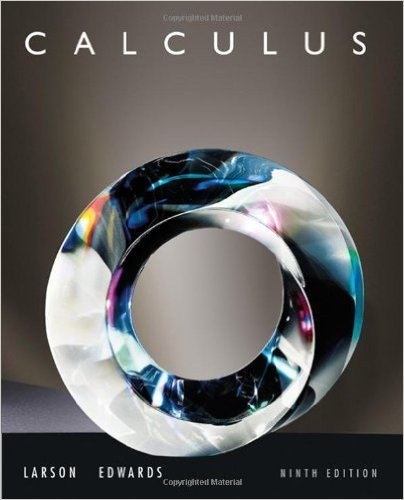×
×

# Solutions for Chapter 5.4: Exponential Functions: Differentiation and Integration## Full solutions for Calculus | 9th Edition

ISBN: 9780547167022Solutions for Chapter 5.4: Exponential Functions: Differentiation and Integration

Solutions for Chapter 5.4
4 5 0 297 Reviews
25
4
##### ISBN: 9780547167022

This textbook survival guide was created for the textbook: Calculus , edition: 9. Since 152 problems in chapter 5.4: Exponential Functions: Differentiation and Integration have been answered, more than 61678 students have viewed full step-by-step solutions from this chapter. This expansive textbook survival guide covers the following chapters and their solutions. Chapter 5.4: Exponential Functions: Differentiation and Integration includes 152 full step-by-step solutions. Calculus was written by and is associated to the ISBN: 9780547167022.

Key Calculus Terms and definitions covered in this textbook
• Amplitude

See Sinusoid.

• Blind experiment

An experiment in which subjects do not know if they have been given an active treatment or a placebo

• Blocking

A feature of some experimental designs that controls for potential differences between subject groups by applying treatments randomly within homogeneous blocks of subjects

• Cofunction identity

An identity that relates the sine, secant, or tangent to the cosine, cosecant, or cotangent, respectively

• Complex plane

A coordinate plane used to represent the complex numbers. The x-axis of the complex plane is called the real axis and the y-axis is the imaginary axis

• Double inequality

A statement that describes a bounded interval, such as 3 ? x < 5

• Empty set

A set with no elements

• Leibniz notation

The notation dy/dx for the derivative of ƒ.

• Negative linear correlation

See Linear correlation.

• One-to-one rule of logarithms

x = y if and only if logb x = logb y.

• Order of an m x n matrix

The order of an m x n matrix is m x n.

• Ordered pair

A pair of real numbers (x, y), p. 12.

• Polar form of a complex number

See Trigonometric form of a complex number.

• Power function

A function of the form ƒ(x) = k . x a, where k and a are nonzero constants. k is the constant of variation and a is the power.

• Pythagorean identities

sin2 u + cos2 u = 1, 1 + tan2 u = sec2 u, and 1 + cot2 u = csc2 u

• Rational function

Function of the form ƒ(x)/g(x) where ƒ(x) and g(x) are polynomials and g(x) is not the zero polynomial.

• Right triangle

A triangle with a 90° angle.

• Sample standard deviation

The standard deviation computed using only a sample of the entire population.

• Sequence

See Finite sequence, Infinite sequence.

• Square matrix

A matrix whose number of rows equals the number of columns.

×